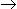# Chemical Engineering - Chemical Reaction Engineering

### Exercise :: Chemical Reaction Engineering - Section 1

41.

For a solid catalysed chemical reaction, the effectiveness of solid catalyst depends upon the __________ adsorption.

 A. physical B. chemical C. both (a) and (b) D. neither (a) nor (b)

Explanation:

No answer description available for this question. Let us discuss.

42.

Pick out the correct statement.

 A. In catalytic reactions, the catalyst reacts with the reactants. B. A catalyst initiates a chemical reaction. C. A catalyst lowers the activation energy of the reacting molecules. D. A catalyst can not be recovered chemi cally unchanged at the end of the chemical reaction.

Explanation:

No answer description available for this question. Let us discuss.

43.

The dimensions of rate constant for reaction 3AB are (l/gm mole)/min. Therefore the reaction order is

 A. 0 B. 1 C. 2 D. 3

Explanation:

No answer description available for this question. Let us discuss.

44.

Pick out the correct statement

 A. Reactions with high activation energies are very temperature sensitive. B. Chemical equilibrium is a static state. C. A photochemical reaction is catalysed by light. D. A chemical reaction occurs when the energy of the reacting molecule is less than the activation energy of the reaction.

Explanation:

No answer description available for this question. Let us discuss.

45.

If the time required to complete a definite fraction of reaction varies inversely as the concentration of the reactants, then the order of reaction is

 A. 0 B. 1 C. 2 D. 3